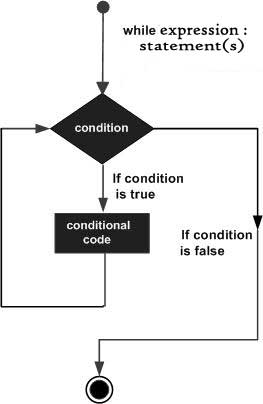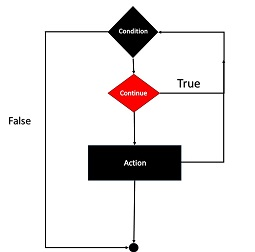# PHP – Loop Types

Loops in PHP are used to execute the same block of code a specified number of times. PHP supports following four loop types.

• for − loops through a block of code a specified number of times.
• while − loops through a block of code if and as long as a specified condition is true.
• do…while − loops through a block of code once, and then repeats the loop as long as a special condition is true.
• foreach − loops through a block of code for each element in an array.

We will discuss about continue and break keywords used to control the loops execution.

## The for loop statement

The for statement is used when you know how many times you want to execute a statement or a block of statements.### Syntax

```for (initialization; condition; increment){
code to be executed;
}
```

The initializer is used to set the start value for the counter of the number of loop iterations. A variable may be declared here for this purpose and it is traditional to name it \$i.

### Example

The following example makes five iterations and changes the assigned value of two variables on each pass of the loop −

```<html>
<body>

<?php
\$a = 0;
\$b = 0;

for( \$i = 0; \$i<5; \$i++ ) {
\$a += 10;
\$b += 5;
}

echo ("At the end of the loop a = \$a and b = \$b" );
?>

</body>
</html>```

This will produce the following result −

```At the end of the loop a = 50 and b = 25
```

## The while loop statement

The while statement will execute a block of code if and as long as a test expression is true.

If the test expression is true then the code block will be executed. After the code has executed the test expression will again be evaluated and the loop will continue until the test expression is found to be false.### Syntax

```while (condition) {
code to be executed;
}
```

### Example

This example decrements a variable value on each iteration of the loop and the counter increments until it reaches 10 when the evaluation is false and the loop ends.

```<html>
<body>

<?php
\$i = 0;
\$num = 50;

while( \$i < 10) {
\$num--;
\$i++;
}

echo ("Loop stopped at i = \$i and num = \$num" );
?>

</body>
</html>```

This will produce the following result −

```Loop stopped at i = 10 and num = 40
```

## The do…while loop statement

The do…while statement will execute a block of code at least once – it then will repeat the loop as long as a condition is true.

### Syntax

```do {
code to be executed;
}
while (condition);
```

### Example

The following example will increment the value of i at least once, and it will continue incrementing the variable i as long as it has a value of less than 10 −

```<html>
<body>

<?php
\$i = 0;
\$num = 0;

do {
\$i++;
}

while( \$i < 10 );
echo ("Loop stopped at i = \$i" );
?>

</body>
</html>```

This will produce the following result −

```Loop stopped at i = 10
```

## The foreach loop statement

The foreach statement is used to loop through arrays. For each pass the value of the current array element is assigned to \$value and the array pointer is moved by one and in the next pass next element will be processed.

### Syntax

```foreach (array as value) {
code to be executed;
}
```

### Example

Try out following example to list out the values of an array.

```<html>
<body>

<?php
\$array = array( 1, 2, 3, 4, 5);

foreach( \$array as \$value ) {
echo "Value is \$value <br />";
}
?>

</body>
</html>```

This will produce the following result −

```Value is 1
Value is 2
Value is 3
Value is 4
Value is 5
```

## The break statement

The PHP break keyword is used to terminate the execution of a loop prematurely.

The break statement is situated inside the statement block. It gives you full control and whenever you want to exit from the loop you can come out. After coming out of a loop immediate statement to the loop will be executed.### Example

In the following example condition test becomes true when the counter value reaches 3 and loop terminates.

```<html>
<body>

<?php
\$i = 0;

while( \$i < 10) {
\$i++;
if( \$i == 3 )break;
}
echo ("Loop stopped at i = \$i" );
?>

</body>
</html>```

This will produce the following result −

```Loop stopped at i = 3
```

## The continue statement

The PHP continue keyword is used to halt the current iteration of a loop but it does not terminate the loop.

Just like the break statement the continue statement is situated inside the statement block containing the code that the loop executes, preceded by a conditional test. For the pass encountering continue statement, rest of the loop code is skipped and next pass starts.### Example

In the following example loop prints the value of array but for which condition becomes true it just skip the code and next value is printed.

```<html>
<body>

<?php
\$array = array( 1, 2, 3, 4, 5);

foreach( \$array as \$value ) {
if( \$value == 3 )continue;
echo "Value is \$value <br />";
}
?>

</body>
</html>```

This will produce the following result −

```Value is 1
Value is 2
Value is 4
Value is 5```